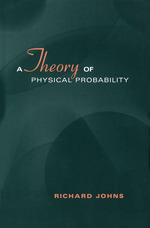Have library access? Log in through your library# A Theory of Physical Probability

RICHARD JOHNS
Copyright Date: 2002
https://doi.org/10.3138/9781442670501
Pages: 304
https://www.jstor.org/stable/10.3138/9781442670501

## Table of Contents

1. Front Matter
(pp. i-iv)
2. Table of Contents
(pp. v-2)
3. 1 Introduction
(pp. 3-8)

There is, at present, no satisfactory theory of physical probability, or chance. In this book I offer such a theory, called the causal theory of chance (or CTC for short). The termchancehas had a number of different meanings, but is now regularly used to refer to the kind of probability that is inherent in some physical systems. The half-life of a nucleus, for instance, is defined as the length of time in which the probability of decay is ½. The use of the term ‘probability’ here has nothing to do with any weight of evidence or degree of...

4. 2 Logic and Probability
(pp. 9-52)

The main aim of this chapter is to argue that there is such a thing as logical probability. The conception of logical probability I defend is somewhat different from previous accounts, however, so why do I call it ‘logical probability’? The essential notion of logical probability, as pioneered by Keynes (1921), is captured by the following three ideas:

1 As the name suggests, logical probabilities are probabilities that are, in some sense, part of logic.

2 A logical probability is a degree of entailment of one proposition by another. Thus, a logical probability represents a logical relation between two propositions....

5. 3 Causation and Determination
(pp. 53-83)

The purpose of this chapter is to investigate the relations of causation and determination, and in particular to argue that a cause need not determine its effects. This work is needed as part of the theoretical foundation for the causal theory of chance, which will be stated in the next chapter. A complete analysis of determination is offered that is similar to standard accounts. No analysis of causation is given, however, as I hold that causation must be taken as primitive. Thus, my view is very much in the same vein as that of Anscombe (1971), and indeed this chapter...

6. 4 Physical Chance
(pp. 84-108)

In this chapter I shall use the ideas developed in chapters 2 and 3 to state the causal theory of chance. There is nothing new about the basic idea, which is similar to a previous proposal,¹ but there are some innovations in matters of detail.

Like Lewis (1980: 109), I think that Miller’s Principle² shows us how to proceed in giving a theory of chance. Miller’s Principle, in its simplest form, states that the epistemic probability of some event A, given only that its physical chance isp,is alsop.Thus we have

If one knows that the chance...

7. 5 Classical Stochastic Mechanics
(pp. 109-147)

As stated in chapter 1, I think an account of physical chance should prove its mettle by being useful in stochastic physics. Physicists do not often say that, in order to understand some aspect of a physical theory, one must first grasp von Mises’s or Popper’s interpretation of probability! An analysis of chance that does at least engage certain problems in physics is surely preferable to one that is independent of them. The remaining chapters are an attempt to show that the causal theory of chance is indeed useful, if not indispensable, in understanding some aspects of physical theory.

In...

8. 6 Correlation
(pp. 148-187)

The topic of probabilistic correlation has become important, and puzzling, due to its central role in quantum theory. Einstein, Podolsky, and Rosen (1935) showed that the rules of quantum mechanics predict correlations between measurement results that seem to require ‘hidden variables,’ that is, physical quantities not represented in the quantum wave function. The hope of explaining these correlations using local hidden variables seemed to be quashed decisively by two results, however, one mathematical and one experimental. The mathematical result was Bell’s theorem (Bell 1964), which showed, within certain assumptions, that any local hidden-variable theory must differ from quantum mechanics in...

9. 7 The State Vector
(pp. 188-232)

In the previous chapter on correlation we saw that the chance function in quantum mechanics does not always satisfy predictive locality. I offered an explanation for why this might be so, but did not give any interpretation of the quantum-mechanical formalism. In this chapter I will develop a theory of what the quantum state vector represents. I shall look at the two main views on this, that (1) it models some physical field, as Bohm, Everett, and R. Penrose maintain, and that (2) it represents ‘knowledge,’ or ‘information’ about the system. My view, perhaps surprisingly, is that both (1) and...

10. 8 Conclusion
(pp. 233-234)

This book covers a wide range of topics, so one may wonder what the overall message is. I think the main theme is that there cannot be a sharp separation between the study of probability by philosophers and the work of physicists. I have argued, in chapters 5 to 7, that certain problems of theoretical physics are greatly illuminated by a correct philosophical understanding of probability and its attendant notions. Moreover, though it may not be apparent in reading the book, the study of physical problems was of crucial importance in formulating the ‘philosophical’ analysis of chance in chapters 2...

11. Notes
(pp. 235-244)
12. Bibliography
(pp. 245-252)
13. Index
(pp. 253-259)